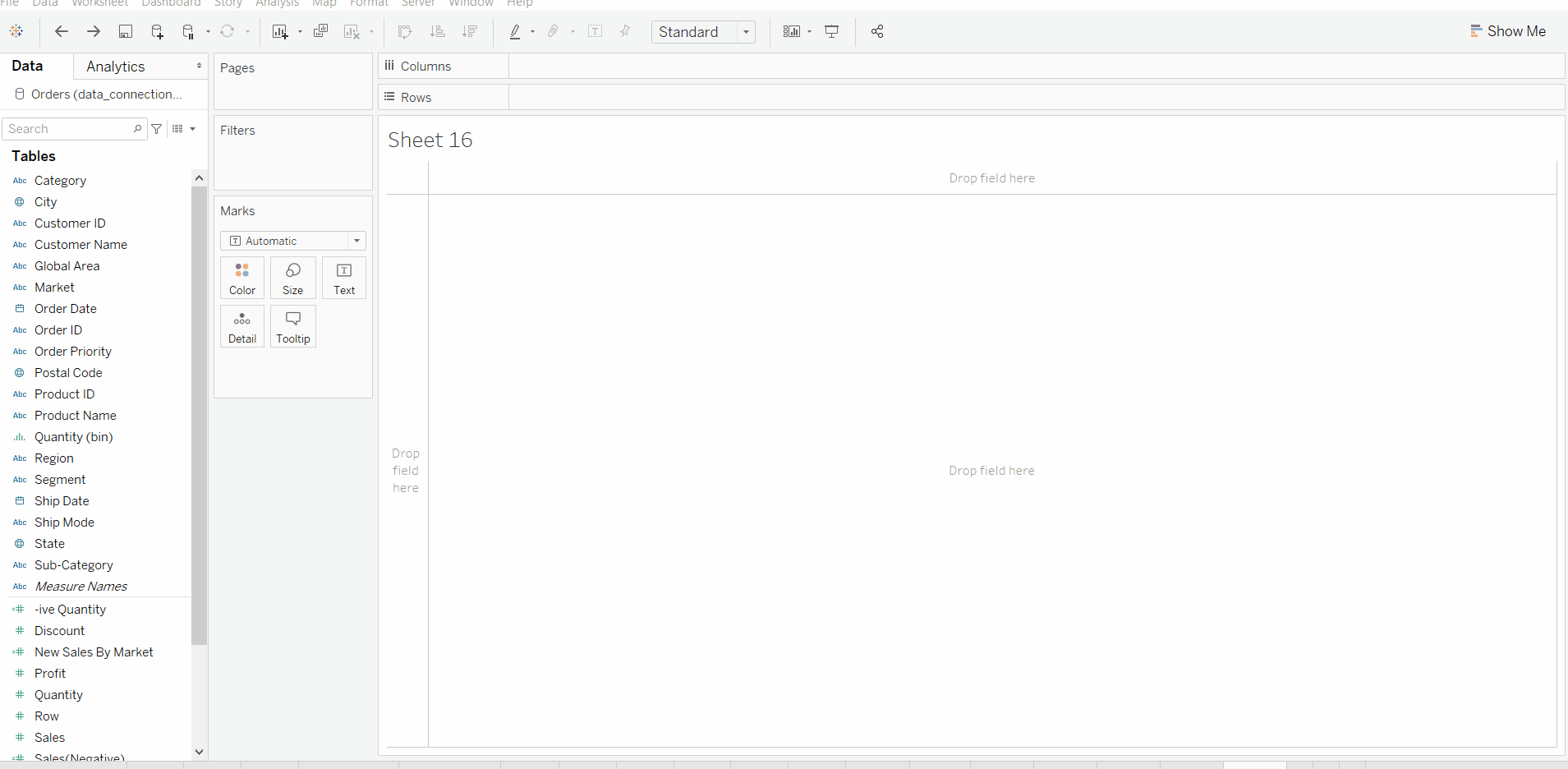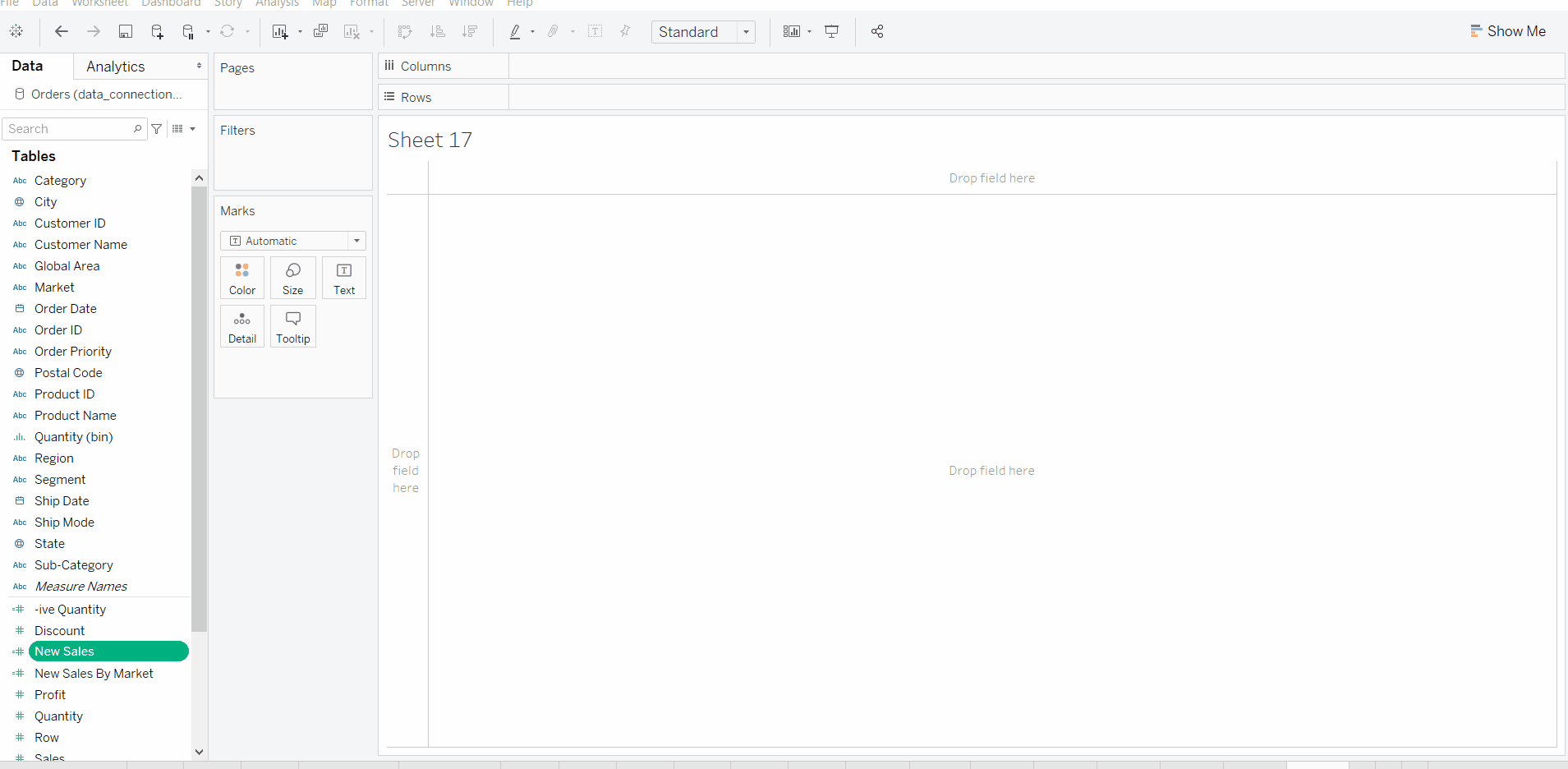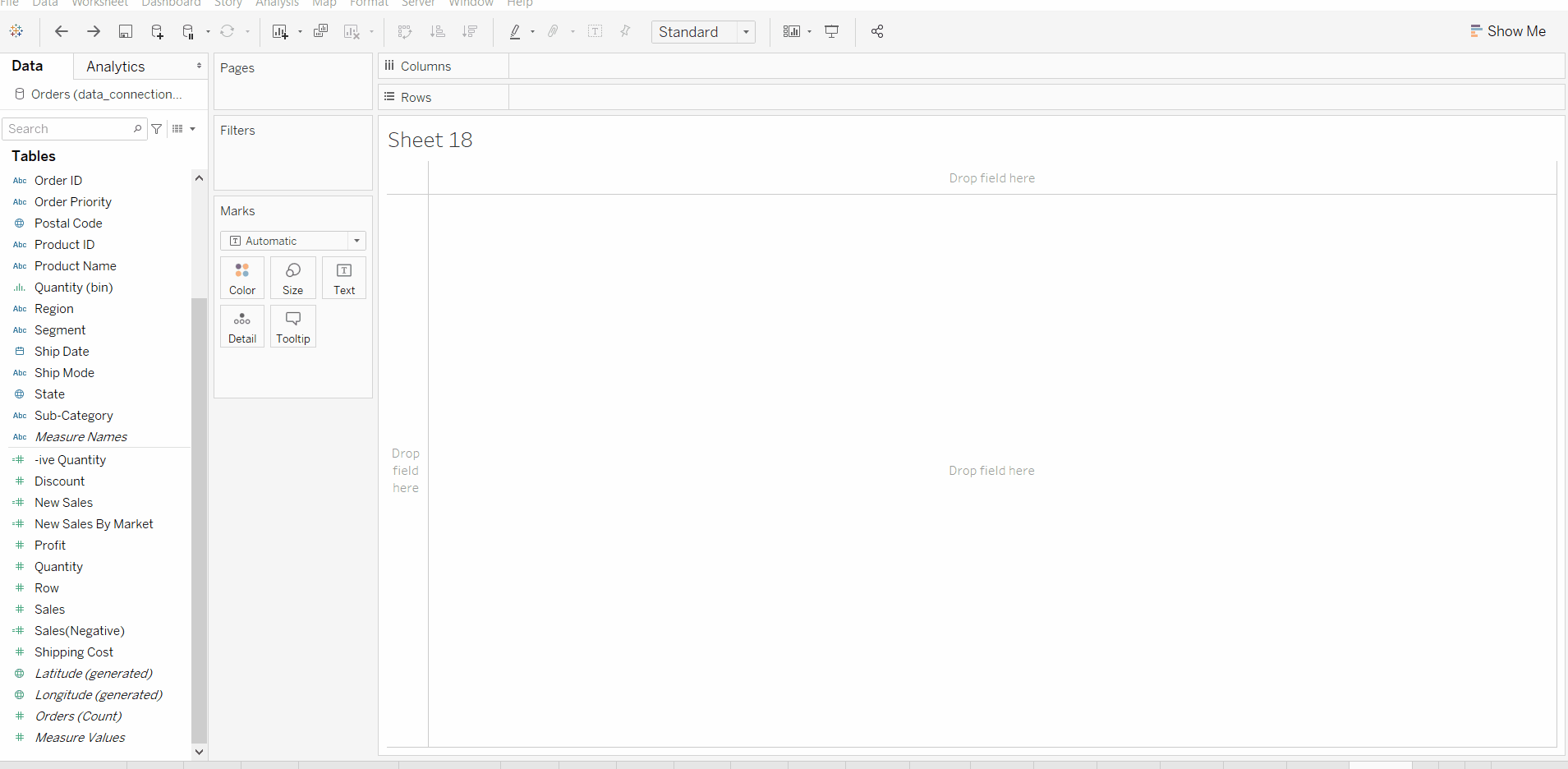Related Articles

# IF Function in Tableau

• Last Updated : 24 Oct, 2020

In this article, we will learn about if function and its use in Tableau. For this first look into two terms:

• Tableau: Tableau is a very powerful data visualization tool that can be used by data analysts, scientists, statisticians, etc. to visualize the data and get a clear opinion based on the data analysis. Tableau is very famous as it can take in data and produce the required data visualization output in a very short time.
• IF Function: Tableau If Function is one of the foremost useful decision-making functions. If the function tests the condition and depending upon the condition result, it’ll return the output.

How IF function works:

• It starts with “IF” function evaluating the 1st logical expression corresponding to set/sequence of values and when the logical expression becomes True, it returns the respective specified value as result.
• If the first doesn’t match then it moves to another if i.e ELSEIF and checks the same as for IF.
• If in logical expressions no match is found then the value of default i.e; ELSE return expression is used.

Syntax of IF Function:

This function checks the given <expression> and returns the corresponding value as a result. The syntax is :

```IF <Expression1> THEN <Statement1>
ELSEIF <Expression2> THEN <Statement2>
ELSEIF <Expression3> THEN <Statement3>
.....
ELSEIF <ExpressionN> THEN <StatementN>
ELSE <Statement>
END
```

Dataset used in the given examples is Dataset.

Example 1: (IF-END)

• In this example, we simply create a new calculated field by using the IF function on a field.
• View a new calculated field.
• Use in Visualization.
• It has a drawback that creates null values in case of the false condition.Example 2: (IF-ELSE-END)

• In this example, we simply edit that previously calculated field by using the IF-ELSE function in the same field.
• View a new calculated field.
• Use in Visualization.
• It overcomes a drawback that creates null values in case of the false condition.Example 3: (IF-ELSEIF-ELSE-END)

• In this example, we simply create a new calculated field by using the IF-ELSEIF-ELSE function on a field.
• View a new calculated field.
• Use in Visualization.My Personal Notes arrow_drop_up